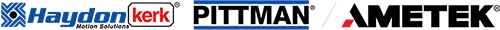In many devices lead screw assemblies are used to convert motion from rotary to linear and vice-versa. Historically, these assemblies have had poor efficiencies and relied on grease for improved performance. Over the past few decades the advent of engineered polymers and new manufacturing capabilities have changed the game for the conventional lead screw assembly making it a powerful solution for motion based design challenges. At first glance lead screw assemblies seem rudimentary, but they are designed to perform a very specific function, and even with the latest developments in materials and manufacturing processes having a basic understanding of how lead screws operate can be the difference between a successful design and catastrophic failure.

To help explain the theory of operation behind lead screws let’s first consider the operation of a basic ramp. The illustration in Figure 1 below shows a person pushing a box up a ramp to raise it from one level to the next. The ramp serves to lift against the force of gravity by transmitting a portion of the person’s horizontal push force into a vertical force. In addition to gravity and the person’s push force there is also a normal force from the ramp and a frictional force between the box and the ramp. Friction is drawn as bi-directional because it always resists the direction of motion which in this case could be up or down the ramp. The frictional force (Ff) is the product of the coefficient of friction between the two sliding surfaces (box and ramp) and the normal force (Fn). In this example there are three factors that will determine how much push force is needed to move the box up the ramp; ramp angle alpha (α), the magnitude of the load, and the coefficient of friction between the box and the ramp. As the ramp angle alpha (α) increases the person needs to apply more push force to move the box up the ramp, but they also need to travel less to achieve the same lift. A large enough ramp angle or a low enough coefficient of friction will allow the box to slide down the ramp if there is no force to oppose it (in a lead screw this phenomenon is known as backdriving).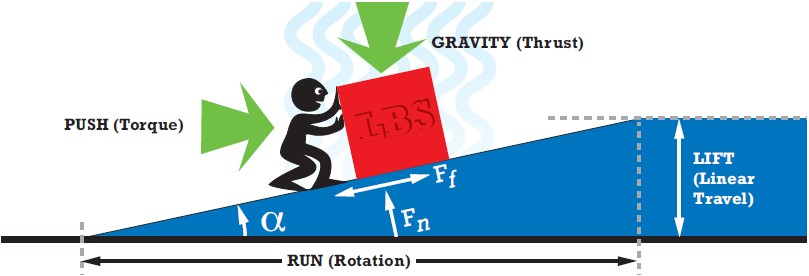Figure 1: Ramp Illustration.

The function of a lead screw is to convert rotational motion to linear motion, or rotational force (torque) to linear force (thrust). For conceptual understanding, the thread form geometry of the lead screw can be ignored which simplifies the screw to a ramp that is wrapped around a cylinder, thus creating a helix. In this case the lift becomes the linear motion component of the screw, and run becomes the rotation of the screw as noted in Figure 1. The lead of the screw is defined as the linear distance traveled per rotation of the screw. The gravity component of the diagram becomes the total thrust force provided by the nut traveling on the screw. The person’s push force becomes the torque input that drives the load and alpha becomes the lead angle of the screw.

Converting the linear thrust and speed into torque and rotational speed is achieved by Equations 1 and 2. Varying the lead will affect both the required torque and speed of the drive input to achieve performance goals. Altering the efficiency will affect the drive torque as can be demonstrated by Equation 1. It is possible to change the lead screw efficiency while keeping the same diameter and lead by altering the coefficient of friction in the assembly. This is commonly done by changing the nut material or adding a coating to the screw.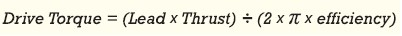Equation 1Equation 2

Proper mounting and alignment of the screw as well as guidance of the load are critical. The mounting configuration contributes to the maximum allowable rotational speed. This can be determined by the simplified critical speed calculation of the screw as shown in Equation 3 which assumes a screw material with a modulus of Elasticity of 28 Mpsi (such as 303 stainless). The mounting factor is one of four unique mounting methods as described in Table 1.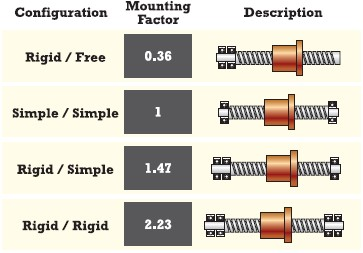Table 1: Mounting Configurations.

Typically the rotational speed of an application is limited to 75% of the critical speed calculated to help account for minor misalignment and manufacturing tolerances. Exceeding the critical speed can cause an unstable condition which can damage the lead screw assembly and other components linked to it.
When using a lead screw assembly with a polymer nut the material’s pressure velocity rating (PV) will need to be considered. Pressure velocity is a property that helps define how much heat generation the material can withstand. The generated heat is caused by the friction between the screw and the nut which is directly related to the thrust force and rotational speed. Exceeding the PV can cause rapid failure of the thread system in a lead screw assembly. There are several ways to reduce the PV of an application such as decreasing the load, decreasing the linear speed, or increasing lead as can be demonstrated by the simplified PV calculation shown in Equation 4.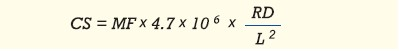Equation 3:

 Where: CS= Critical Speed in RPM MF = Mounting Factor RD = Root Diameter of the Lead Screw in Inches L = Length between Supports in Inches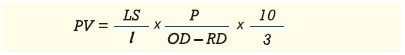Equation 4:

 Where: PV = Pressure Velocity in psi fpm LS = Linear Speed in Inches per Second l = Lead in inches P = Load in Pounds OD = Outside Diameter of the Lead Screw in Inches RD = Root Diameter of the Lead Screw in Inches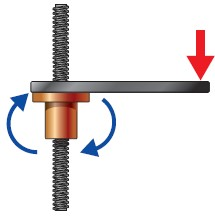Figure 2a: Unguided lead screw axis.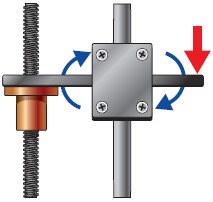Figure 2b: Guided lead screw axis.Now that we’ve covered some of the theory let’s put it to practice on an example.Equation 5Equation 6Equation 7

With the standard materials of a 303 SS lead screw and acetal based nut the manufacturer specifies an efficiency of 73.1% for a 0.250 inch diameter screw with a 0.500 inch lead. Applying this information as well as the required thrust to Equation 1 results in a required drive torque of 43.5 oz-in as shown in Equation 8 below. This is within the motors capability of 60 oz-in and provides some torque overhead.Equation 8

Now that a diameter and lead have been selected that meet the speed and thrust requirements of the application when driven by the selected motor the next step is to verify the support configuration and material selection. From the manufacturer’s specification sheet the root diameter of the lead screw is 0.169 inches. With this additional information, and Equation 3, the critical speed of the lead screw configuration is calculated to be 3,103 RPM as demonstrated by Equation 9. To account for misalignment and manufacturing tolerances the critical speed limit is typically set to 75% of the calculated value. Equation 10 shows the critical speed to be 2,327 RPM with a safety factor applied. The maximum speed requirement of the application of 480 RPM is well within the limiting critical speed of the screw configuration.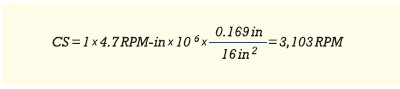Equation 9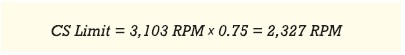Equation 10

The desired material for the nut used in this application is acetal based and has a pressure velocity limit of approximately 12,000 psi fpm. Using Equation 4 the pressure velocity with the configuration selected is 8,230 psi fpm as shown in Equation 11. With the basic parameters covered the 0.25 inch diameter screw with a 0.500 inch lead will meet the needs of the application when coupled to the available motor. Another important piece of information in the design of this axis is that the lead is efficient enough to backdrive, and to keep the load from falling under its own weight when power is removed from the motor, a brake will need to be implemented.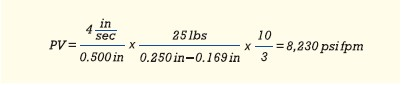Equation 11

There are other topics to consider when designing a lead screw driven axis, but the concepts discussed cover the basics and provide a solid foundation to design a successful lead screw driven axis of motion. Understanding how to properly implement a lead screw into a design will improve the chances of success by avoiding potential design pitfalls. When used properly, lead screws are a powerful tool which can be used in many design solutions that not only solve the need to convert rotary to linear motion, but also significantly improve productivity and motion control precision in large-to-micro scale advanced technology applications.